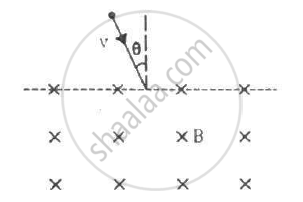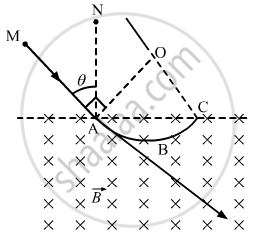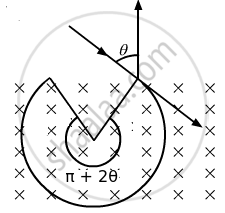Advertisement Remove all ads

# A Particle of Mass M and Positive Charge Q, Moving with a Uniform Velocity V, Enters a Magnetic Field B, as Shown in the Figure. - Physics

Sum

A particle of mass m and positive charge q, moving with a uniform velocity v, enters a magnetic field B, as shown in the figure. (a) Find the radius of the circular arc it describes in the magnetic field. (b) Find the angle subtended by the arc at the centre. (c) How long does the particle stay inside the magnetic field? (d) Solve the three parts of the above problem if the charge q on the particle is negative.Advertisement Remove all ads

#### Solution

Given:-

Mass of the particle = m

Positive charge on the particle = q

Uniform velocity of the particle = v

Magnetic field = B

(a) The radius of the circular arc described by the particle in the magnetic field:-

We know

r = (mv)/(qB)

(b)The angle subtended by the arc at the centre:-

Line MAB is tangent to arc ABC, so the angle described by the charged particle,

∠MAO = 90°

Now, ∠NAC = 90°

OAC = OCA = θ

[by geometry]

Then, AOC = 180° − (θ + θ) (By angle-sum property of a triangle)

= π − 2θ

(c) The time for which the particle stay inside the magnetic field:-

Distance covered by the particle inside the magnetic field,

l = rθ

t = 1/v = m/(qB)(pi - 2theta)(U sing r = (mv)/(qB))

(d) If the charge q on the particle is negative, then(i) Radius of circular arc, r = (mv)/(qB)

(ii) The centre of the arc will lie within the magnetic field. Therefore, the angle subtended by the arc = π + 2θ

(iii) Similarly, the time taken by the particle to cover the path inside the magnetic field = m/(qB)(pi + 2theta)

Concept: Force on a Moving Charge in Uniform Magnetic and Electric Fields
Is there an error in this question or solution?
Advertisement Remove all ads

#### APPEARS IN

HC Verma Class 11, Class 12 Concepts of Physics Vol. 2
Chapter 12 Magnetic Field
Q 38 | Page 233
Advertisement Remove all ads
Advertisement Remove all ads
Share
Notifications

View all notifications

Forgot password?